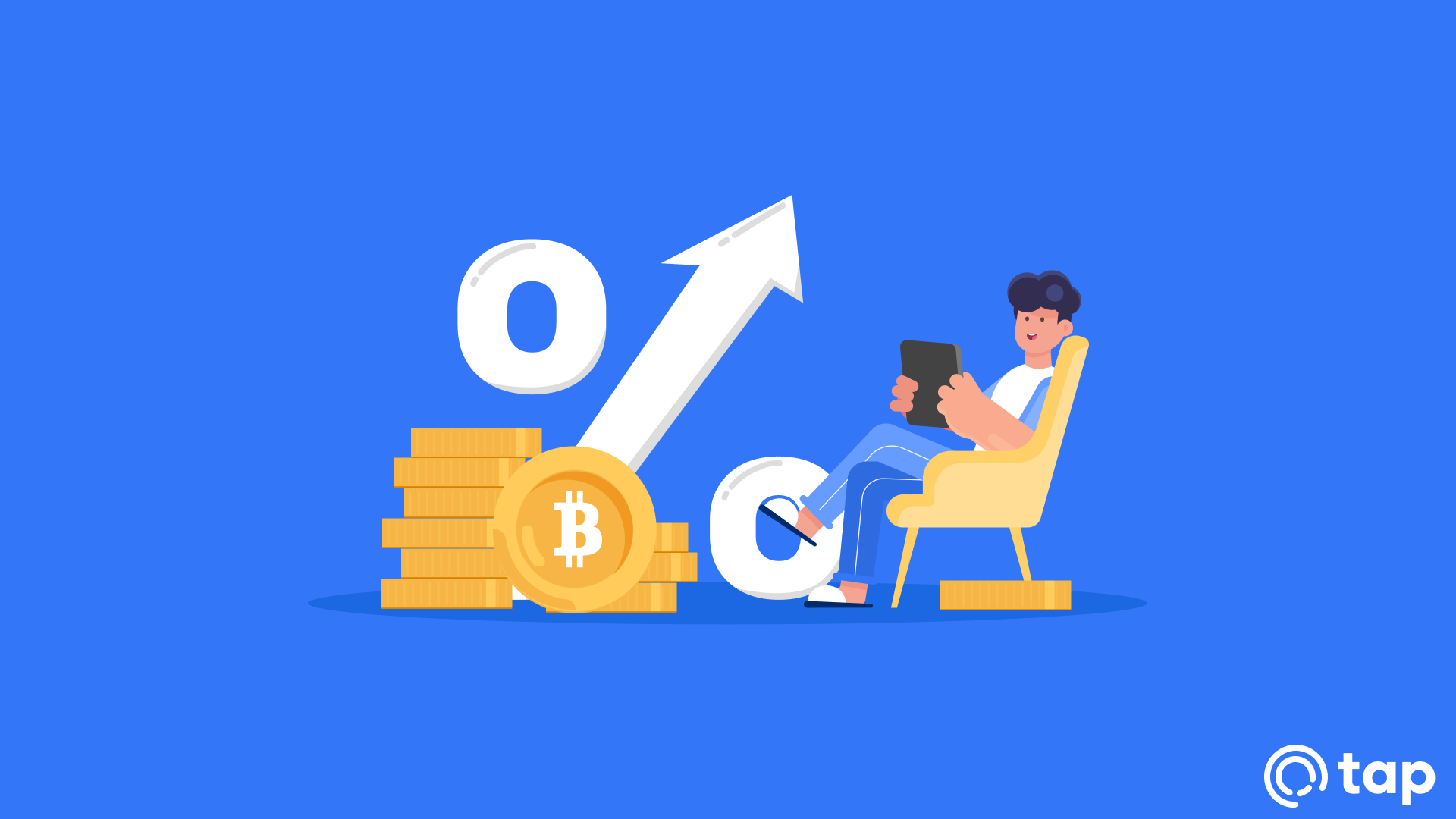# What Is Interest?

Interest is the price attached to borrowing money or the price you charge to lend money out. Interest is often reflected or calculated as the annual percentage of the loan amount over its pay-off amount.

There are three main types of interest, including but not limited to simple interest, accrued interest, and compounding interest such as the one offered by Tap Earn. Interest is usually quoted as an annual rate, as stated, but can be calculated for longer or shorter periods than a year. The percentage rate applied to interest charged on loans is referred to as an interest rate.

Some of the main factors affecting interest rates can be seen as the general inflation rate, length of time the money is borrowed out, liquidity, as well as the risk of default. The amount the lender charges for interest depends on many factors. Things that can impact interest rates include the type of loan, expected inflation, length of time, credit history, etc.